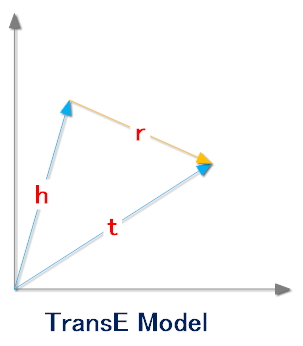## TransE 作用

h表示头实体
r表示关系
t表示尾实体


h 表示头实体对应的向量
r 表示关系对应的向量
t 表示尾实体对应的向量


[0.01, 0.04, 0.8, 0.32, 0.09, 0.18]


## TransE的基本思想TransE模型认为一个正确的三元组的embedding向量(h,r,t)会满足公式:h+r=t

## TransE的目标函数

$$||\textbf{x}||2 =\sqrt{\sum{i=1}^Nx_i^2}$$

Euclid范数（欧几里得范数，常用计算向量长度），即向量元素绝对值的平方和再开方。

$$min\sum{(h,r,t)\in\Delta} \sum{(h^{‘},r^{‘},t^{‘})\in\Delta}[\gamma + f(h,r,t) - f(h^{‘},r^{‘},t^{‘})]_+$$

$\Delta$ 表示正确的三元组集合
$\Delta^{‘}$表示错误的三元组集合
$\gamma$ 表示正负样本之间的间距，是一个常数
$[x]_+$ 表示max(0,x)

||h||≤1,||r||≤1,||t||≤1。## transE评价方法

### 基本的评价过程

• 将一个正确的三元组a中的头实体或者尾实体，依次替换为整个知识库中的所有其它实体，也就是会产生n个三元组。
• 分别对上述n个三元组计算其能量值，在transE中，就是计算h+r-t的值。这样可以得到n个能量值，分别对应上述n个三元组。
• 对上述n个能量值进行升序排序。
• 记录三元组a的能量值排序后的序号。
• 对所有的正确的三元组重复上述过程。
• 每个正确三元组的能量值排序后的序号求平均，得到的值我们称为Mean Rank。
• 计算正确三元组的能量排序后的序号小于10的比例，得到的值我们称为Hits@10。

### 改进

• 将一个正确的三元组a中的头实体或者尾实体，依次替换为整个知识库中的所有其它实体，也就是会产生n个三元组。
• 分别对上述n个三元组计算其能量值，在transE中，就是计算h+r-t的值。这样可以得到n个能量值，分别对应上述n个三元组。
• 对上述n个能量值进行升序排序。
• 记录三元组a的能量值排序后的序号k。
• 如果前k-1个能量对应的三元组中有m个三元组也是正确的，那么三元组a的序号改为k-m。
• 对所有的正确的三元组重复上述过程。
• 每个正确三元组的能量值排序后的序号求平均，得到的值我们称为Filtered Mean Rank。
• 计算正确三元组的能量排序后的序号小于10的比例，得到的值我们称为Filtered Hits@10。